Single Molecule Studies Using Magnetic Tweezers

Introduction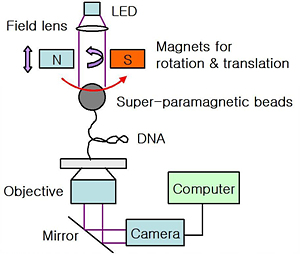Fig. 1: Schematic view of the setup (not to scale)

Over the past ten years, a variety of single molecule nano-manipulation techniques (optical tweezers, atomic force microscopes, magnetic traps) have been developed and used to investigate the mechanical response to force of different biopolymers including DNA, RNA and proteins [1-9]. Among the techniques, magnetic tweezers (MT) is very useful method that has many advantages for biophysical research. First of all, MT is very efficient to produce rotational force as well as translational force (typically pN~nN) with just an extremely simple setup, while twisting DNA using optical tweezers requires a rather complex experiment . Another advantage is that MT gives no damage to the observing bio-molecules, whereas strong laser power for optical tweezers sometimes results in photodamage to the molecules. Last but not least, MT is relatively much cheaper than other tools to construct or purchase. Although MT may not be proper for the experiment that requires highly sensitive control of the molecules as optical tweezers can do, it is more useful than other techniques in some aspects as will be introduced in the next sections. In this report, the principle of MT and its application to a biophysical problem, e. g. the measurement of the elastic properties of DNA, are briefly described.

Physics of Magnetic Tweezers

Basic Concept

MT is composed of a couple of magnets which produces a strong magnetic field gradient. We can change the applied force by moving the relative position of the magnets above a bead. We consider a paramagnetic bead which is trapped by the magnetic field gradient as shown in Fig. 1. The applied magnetic force on the paramagnetic bead is given by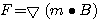where m is a magnetic dipole moment of the bead and B is an applied magnetic field . For strong magnetic fields, the magnetization (M = m/V) of a magnetic bead saturates and approaches the maximum value (Mmax) due to the properties of superparamagnetic materials. Then, the force becomes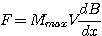Therefore, the direction of the applied force is upward and the magnitude can be considered constant for a given position of the magnets because the bead size (~um) is usually much smaller than the magnets (~mm). Besides, it is very simple and easy to exert not only a constant force but also a torque to biomolecules using this magnetic trapping method. Just simple rotation of the magnets using a rotational motor can produce a torque to the molecules. Fig. 1 shows a DNA sample whose one end is attached to a magnetic bead and another end is tethered on a coverglass using a strong chemical binding, such as streptavidin-biotin. Here, DNA can be stretched or twisted simply by moving downward or rotating the magnets. Now, the remaining problem for the quantitative analysis of the magnetic manipulation is how to measure the applied force.

How to measure the applied force?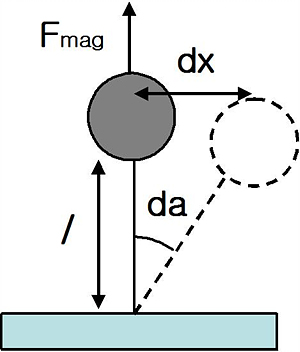Fig. 2: Principle of force measurement 

Fig. 2 shows how to measure the force in a consistent way. We consider a magnetic bead which is in solution and moves in a Brownian motion due to the surrounding water molecules around the bead. The vertical magnetic force applied to the bead stretches the DNA molecule. Then, the trapped bead can move left and right, and the transverse movement satisfies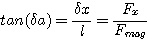Here, l is a DNA extension length, δx is a particle transverse fluctuation, δα is a fluctuation angle, Fmag is a magnetic force, and Fx is a transverse direction force induced by the brownian force. This inverted pendulum behaves like a spring motion, thus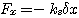where ks is a spring constant. According to the equipartition theorem,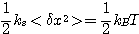where kB is Boltzman constant, T indicates absolute temperature, and ⟨ ... ⟩ means time average. Therefore, the above equations permits the measurement of the stretching force as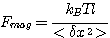Now, we can precisely measure the applied force in real time, if we can acquire the values for T, l and ⟨δx2.

How to measure ⟨δx2⟩ and l ?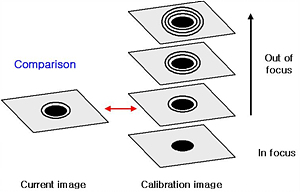Fig. 3: Principle of the vertical length l measurement

First, the measurement of T is trivial. Second, ⟨δx2 can be measured from the image data through the camera and computer. We can compute the displacement of centroid position of the trapped bead by using data analysis procedure, such as intensity correlation function between each frame and its interpolation for the maximum value. This method is useful especially when it is required to find the subpixel position of the centroid from the low resolution pixel image data. Finally, the vertical length l can be measured by using the fact that the bead positioned out of focus makes diffraction ring around the image as shown in Fig. 3. At a given time, the current bead image is compared with the calibration images which were previously constructed. Interpolating the image from the calibrated images yields the optimal vertical length. Up to now, the basic concept of MT and the method to measure the applied force have been introduced. A large number of interesting biophysical applications resulted from using MT.

Twisting DNA

Different with most polymers, DNA is very sensitive to torsion because of its double-helical structure. This particular feature explains why DNA molecules in vivo are twisted, and how negatively supercoiled DNA can locally denature for transcription. Linking number (Lk) is a quantity to show the topological structure of DNA, which is defined by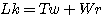where Tw is twist number (# of helical repeats) and Wr is writhe number (# of braided string). When there is no torsion, the quantities become Lk0=Tw0+Wr0 with Wr0=0. In order to understand the supercoiling, we define superhelical density denoted by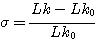DNA of most organisms is negatively supercoiled (m), indicating that it is underwound. Fig. 4 clearly shows the chiral nature of DNA (right handed helix). When considering a worm-like chain model of DNA , total energy of DNA can be expressed by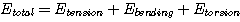where Etension is the energy for stretching, Ebending is for bending, and Etorsion is for twisting. Keep in mind that the negative charge of DNA is screened by a positive ion in 10mM PB, thus the reduced charge repulsion allows easy conformation of braided/twisted structure. Also, it is initially stretched by a moderate force (F=1pN) before inducing a positive/negative torque. When starting the rotation of the bead to the positive direction (right hand), the extension is found to be initially unchanged and the additional energy is stored as torsion. However, as continuing the twist, it is found that the end-to-end length linearly decreases after a certain number of turns. That is because the rotation of DNA to the positive direction after a critical value significantly increases B due to the initial right handed helical structure of DNA. Thus, the twist energy is transferred into the bending energy, and the DNA gradually conforms braided structure more and more. On the other hand, negative rotation does not change the DNA extension as shown in the left side of the figure. Since the left handed torsion loosens the right handed helix, the DNA does not need to change the conformation to balance the energy between bending and torsion. Despite this chiral nature of DNA, it will conform negative supercoiling structure when it is strongly twisted or if there is no stretching force.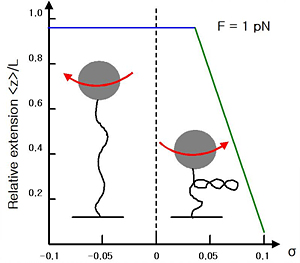Fig. 4: Extension versus supercoiling curve of DNA for 1 pN stretching force in 10 mM PB. 

Concluding remarks

Due to the simple architecture and manipulation MT is one of the most promising and popular biophysical techniques. MT has found wide and powerful use in biophysical systems ranging from the understanding of viscoelastic properties of single DNA and RNA molecules to rheology of soft matter . MT will be more powerful in the near future, if it is combined with highly sensitive detection techniques such as single molecle fluorescence resonance energy transfer (spatial resolution ~ 0.5 nm) as it is recently succeed in the combination with optical tweezers .

Acknowledgement

I appreciate my classmate Sonny Vo for his valuable comment and discussion on the DNA twisting problem.

© 2007 Jong Min Sung. The author grants permission to copy, distribute and display this work in unaltered form, with attribution to the author, for noncommercial purposes only. All other rights, including commercial rights, are reserved to the author.

References

 L. Paterson, M. P. MacDonald, J. Arlt, W. Sibbett, P. E. Bryant and K. Dholakia, "Controlled Rotation of Optically Trapped Microscopic Particles," Science 292, 912 (2001).

 C. Haber and D. Wirtz, "Magnetic Tweezers for DNA Micromanipulation," Rev. Sci. Instrum. 71, 4561 (2000).

 C. Gosse and V. Croquette, "Magnetic Tweezers: Micromanipulation and Force Measurement at the Molecular Level," Biophys. J. 82, 3314 (2002).

 T. R. Strick, J. F. Allemand, D. Bensimon and V. Croquette, "Behavior of Supercoiled DNA," Biophys. J. 74, 2016 (1998).

 C. Bustamante, J. F. Marko, E. D. Siggia and S. Smith. "Entropic Elasticity of λ-phage DNA," Science 265, 1599 (1994).

 Wikipedia, the free encyclopedia <http://en.wikipedipedia.org/wiki/Magnetic-tweezer>

 S. Hohng et al., "Fluorescence-Force Spectroscopy Maps Two-Dimensional Reaction Landscape of the Holliday Junction," Science 318, 279 (2007).

 A. Revyakin, R. H. Ebright and T. R. Strick, "Single-Molecule DNA Nanomanipulation: Improved Resolution Through Use of Shorter DNA Fragments," Nature Method 2, 127 (2005).

 R. T. Pomerantz et al., "A Tightly Regulated Molecular Motor Based Upon T7 RNA Polymerase," Nano L. 5, 1698 (2005).